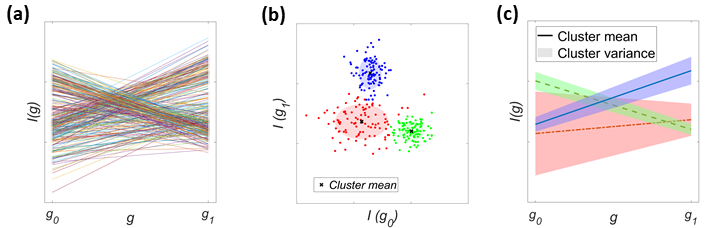# Machine Learning

The massive data that modern facilities generate spanning 3D reciprocal space volumes include ?(104) Brillouin zones (BZ) at rates of ?(102) gigabytes per hour. The sheer quantity of data presents a major challenge in searching for unknown order parameters and their fluctuations.

When the temperature ? is lowered below a certain threshold, the system can give way to an ordered state. Hence the temperature evolution of the X-ray diffraction (XRD) intensity for reciprocal space points qI(q,T) must be qualitatively different if the given reciprocal space point q reflects order parameters or their fluctuations. Tracking the temperature evolution of thousands of Brillouin zones to identify systematic trends and correlations in a comprehensive manner is impossible to achieve manually without selection bias.XTEC is an unsupervised and interpretable ML algorithm that can identify the order parameters from the voluminous data by clustering the temperature series associated with a given q, I(q,T), according to qualitative features in the temperature dependence.At the core of XTEC is a Gaussian Mixture Model (GMM) clustering to identify distinct temperature trajectories. The figure below shows a simplified illustration of GMM clustering behind XTEC.

To cluster distinct I(g) trajectories, given the collection of series {I(g0), I(g1), … ,I(gN-1)} (N=2 in the above figure), the raw trajectories (in panel (a)) can be mapped to a simple Gaussian Mixture Model (GMM) clustering problem on a N-dimensional space (panel (b)). In the above figure, GMM clustering identifies three distinct clusters color-coded as red, blue and green. From the GMM cluster mean and variance (panel (b)), we get the distinct trajectories of I(g) and their variance (panel (c)).

Note that g can be any parameter like temperature, time, energy etc. Hence apart from temperature series data, you can adapt XTEC to analyze any other parametric dependence like time or energy series data.

The following contains a summary of the steps involved in a typical X-TEC analysis. You can follow the steps listed in a Jupyter notebook tutorial (XTEC_Tutorial.ipynb) in the X-TEC Github repository.